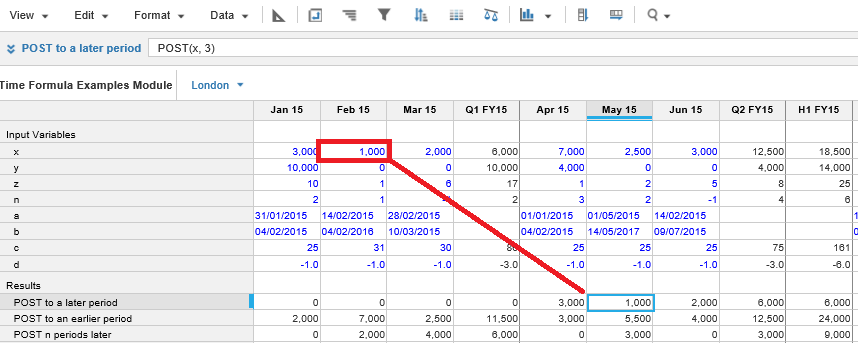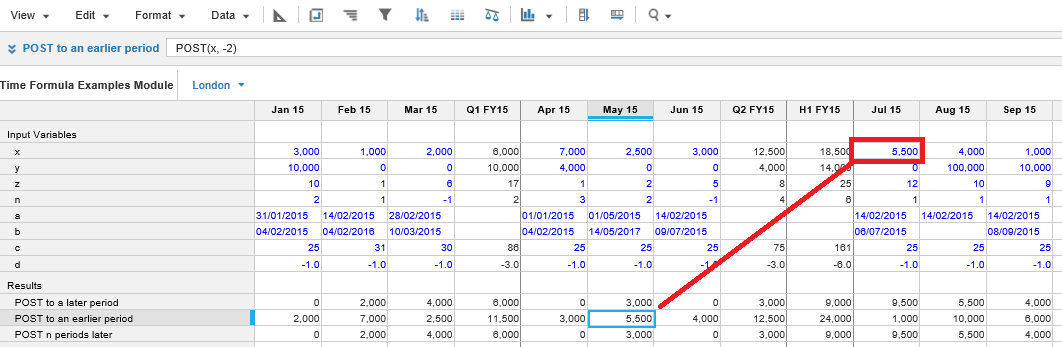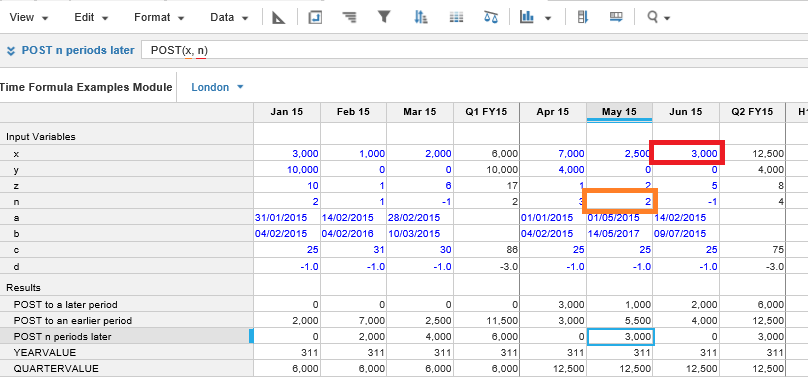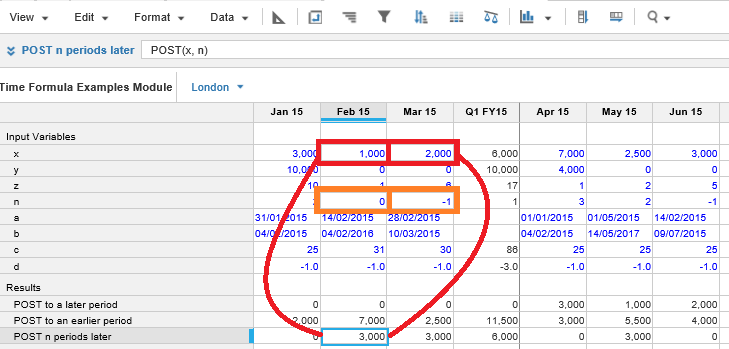1. Calculation functions
2. All Functions
3. Time and Date Functions
4. POSTReturns the value in a line item from a future or previous period based on a positive or negative offset value.

The offset for each period determines where the source value is to be posted to. POST can use a second line item as the offset amount, which could result in more than one source value being posted to a target period.

Since the offset can vary by period, this can be used to add up selected periods in the timescale.

POST only works on number-formatted line items.

## Syntax

`POST(x, n) `

where:

• x: Line item: value to be returned
• n: Offset amount

## Format

Input Format Output Format

x: Line item formatted to a number

n: Number

Number

## Arguments

The function uses the following arguments:

• x: Line item: Numeric
• n: Number: Numeric line item, property, or expression.

## Constraints

The function has the following constraints:

• The offset for each period determines where the source value is posted. Negative value pushes to later periods, positive value retrieves POST earlier periods.
• POST can result in more than one source value being posted to a target period.
• POST is only applicable to numbers.
• The time range providing arguments to a line item must match the time range for the result line item. Any formula that conflicts with this rule will be rejected. For more information, see Search page.
• A non-time series function, used as a sub-expression of this function, must use the same time range as the line item to which the formula is applied. Combining time series functions and non-time series functions with a time range could return an unexpected result or an error message. For more information, see Time Ranges and Time Series Functions page.

## Excel equivalent

• No Excel equivalent

## Example

The formula:

`POST(x, 3) `

returns the value in line item x to 3 time periods in the future.The formula:

`POST(x, -2)`

returns the value in line item x to 2 time periods in the past.The formula:

`POST(x, n)`

returns the value in line item x offset to the value n.The example below shows how two cells are pushed to the same destination cell. The values in row n are editable.The x value in column Mar15 is posted to column Feb 15 in row Post n periods later by the value n in Mar 15.

At the same time, the x value in column Feb15 is posted to cell Feb 15 in row Post n periods later by the value n in Feb 15.